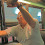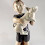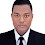## Saturday, October 21, 2017

### Greg's algebra

How much do workers gain from a capital tax cut? This question has reverberated in oped pages and blogosphere, with the usual vitriol at anyone who might even speculate that a dollar in tax cuts could raise wages by more than a dollar. (I vaguely recall more blogosphere discussion which I now can't find, I welcome links from commenters. Greg was too polite to link to it.)

Greg Mankiw posted a really lovely little example of how this is, in fact, a rather natural result.

However, Greg posted it as a little puzzle, and the average reader may not have taken pen and paper out to solve the puzzle. (I will admit I had to take out pen and paper too.) So, here is the answer to Greg's puzzle, with a little of the background fleshed out.

The production technology is $Y=F(K,L)=f(k)L;k\equiv K/L$ where the second equality defines $$f(k)$$. For example $$K^{\alpha}L^{1-\alpha}=(K/L)^{\alpha}L$$ is of this form. Firms maximize $\max\ (1-\tau)\left[ F(K,L)-wL \right] -rK$ $\max\ (1-\tau)\left[ f\left( \frac{K}{L}\right) L-wL \right] -rK$

The firm's first order conditions are $\partial/\partial K:(1-\tau)f^{\prime}\left( \frac{K}{L}\right) \frac{1}{L}L=r$ $(1-\tau)f^{\prime}\left( k\right) =r$ $\partial/\partial L:f\left( \frac{K}{L}\right) -f^{\prime}\left( \frac {K}{L}\right) \frac{K}{L^{2}}L=w$ $f(k)-f^{\prime}(k)k=w.$ Total taxes are $X=\tau\left[ F(K,L)-wL\right]$ so taxes per worker are $x=\tau\left[ f(k)-w\right] =\tau f^{\prime}(k)k.$ Now, let us change the tax rate. The static -- neglecting the change in capital -- cost of the tax change, per worker, is $\frac{dx}{d\tau}=f^{\prime}(k)k.$ To find the change in wages, differentiate that first order condition, $\frac{dw}{d\tau}=\left[ f^{\prime}(k)-f^{\prime\prime}(k)k-f^{\prime }(k)\right] \frac{dk}{d\tau}=-kf^{\prime\prime}(k)\frac{dk}{d\tau}.$ To find the change in capital, differentiate that first order condition, and remember the assumption that the return to capital is fixed at $$r$$, so $$dr/d\tau=0$$ $-f^{\prime}(k)d\tau+(1-\tau)f^{\prime\prime}(k)dk=0$ $\frac{dk}{d\tau}=\frac{f^{\prime}(k)}{(1-\tau)f^{\prime\prime}(k)}.$ Now use this on the right hand side of the $$dw/d\tau$$ equation, $\frac{dw}{d\tau}=-kf^{\prime\prime}(k)\frac{f^{\prime}(k)}{(1-\tau )f^{\prime\prime}(k)}=-\frac{kf^{\prime}(k)}{1-\tau}=-\frac{1}{1-\tau}\frac {dx}{d\tau}.$ Dividing, $\frac{dw}{dx}=-\frac{1}{1-\tau}$ (Greg has a +, since he defined a negative change in the tax rate.) Each dollar (per worker) of static tax losses raises wages by $$1/(1-\tau)$$. It's always greater than one. For $$\tau=1/3$$, each dollar of tax cut raises wages by $1.50. A number greater than one does not mean you're a moron, incapable of addition, a stooge of the corporate class, etc. The example is gorgeous, because all the production function parameters drop out. Usually you have to calibrate things like the parameter $$\alpha$$ and then argue about that. This is not the same as the Laffer curve, which I think causes some of the confusion. The question is not whether one dollar of static tax cut produces more than a dollar of revenue. The question is whether it raises capital enough to produce more than a dollar of wages. This is also a lovely little example for people who decry math in economics. At a verbal level, who knows? It seems plausible that a$1 tax cut could never raise wages by more than $1. Your head swims. A few lines of algebra later, and the argument is clear. You could never do this verbally. You might object though that we use the dynamic wage rise over the static tax loss. However, that (at least in my hands) does not lead to so beautiful a result. Also, the political and blogosphere argument is over how much wages will rise relative to the static tax losses. Moreover, the dynamic tax loss is lower. So Greg's calculation is a lower bound on the rise in wages relative to the true loss in tax revenue. Update: Thanks to a Jason Furman tweet, I was inspired to keep going. Here is the dynamic result: $\frac{dx}{d\tau}=kf^{\prime}(k)+\tau\left[ f^{\prime}(k)+kf^{^{\prime\prime} }(k)\right] \frac{dk}{d\tau}$ We had $\frac{dw}{d\tau}=-kf^{\prime\prime}(k)\frac{dk}{d\tau}$ $\frac{dk}{d\tau}=\frac{f^{\prime}(k)}{(1-\tau)f^{\prime\prime}(k)}$ so $\frac{dx}{dw}=\frac{kf^{\prime}(k)+\tau\left[ f^{\prime}(k)+kf^{^{\prime \prime}}(k)\right] \frac{dk}{d\tau}}{-kf^{\prime\prime}(k)\frac{dk}{d\tau}}$ $\frac{dx}{dw}=-\frac{f^{\prime}(k)(1-\tau)f^{\prime\prime}(k)}{f^{\prime \prime}(k)f^{\prime}(k)}-\frac{\tau\left[ f^{\prime}(k)+kf^{^{\prime\prime} }(k)\right] }{kf^{\prime\prime}(k)}$ $\frac{dx}{dw}=-(1-\tau)-\tau\left[ 1+\frac{f^{\prime}(k)}{kf^{\prime\prime }(k)}\right]$ $\frac{dx}{dw}=-(1-\tau)-\tau\left[ 1+\frac{\alpha k^{\alpha-1}}{\alpha (\alpha-1)k^{\alpha-1}}\right]$ $\frac{dx}{dw}=-(1-\tau)-\tau\left[ 1+\frac{1}{\alpha-1}\right]$ $\frac{dx}{dw}=\frac{\left( \alpha-1\right) \left( \tau-1\right) -\tau\alpha}{\alpha-1}$ $\frac{dx}{dw}=-\frac{1-\tau-\alpha}{1-\alpha}$ Inverting, and using $$\alpha=1/3$$, now$1 in capital tax loss gives rise to $2.00 in extra wages, not just$1.50. Thanks Jason!

Jason goes on to say this "misses much of what matters in tax policy," a point with which I heartily agree. The point of Greg's, and my post, though, was a response to the commentary that anyone that thought that lowering capital taxes could possibly raise wages at all, let alone one for one, let alone more than one for one, was a "liar", evil, stupid, and so forth. Among other things, lowering capital taxes can raise wages, and more than one for one in very simple models. It has lots of other effects which we can discuss. I still like zero, burn the code, burn all the rotten cronyist exemptions, in a revenue neutral reform. But that's for another day.

Update 2: Casey Mulligan's blog is a must read on this issue, both for more intellectual history, and a graphical analysis. Be sure to click Casey's "algebra here" link, or directly here to see how he does this algebra by machine.

Update 3: in response to a correspondent's request for the idea in words: A corporation invests up to the point that the after-tax return on its investment equals the return investors demand to give the corporation capital. So, let us suppose the tax rate is one half. To give investors a 5% return, the corporation must pursue projects that earn a 10% before tax return. Suppose we eliminate this tax. Now, new projects, that offer a return between 5% and 10% become profitable. The company borrows or issues stock, and buys new machines, factories, etc. These new machines and factories make workers more productive. The firm wants to hire more workers to run the new machines. But there are only so many workers available in the economy, and everyone is doing the same thing. Firms bid against each other for the workers, raising wages. Eventually wages rise, so the firm has the same number of workers, but each one is more productive because they have more machines at their disposal. Lowering corporate taxes raises wages.

1.What does the government do with the tax money?

1.That's an opportunity for additional research. Perhaps the government is enforcing property rights and rules of contract to preclude the embezzlement of some of the aggregate output Y to other uses. Yes, that's getting outside of the model, but there might be ways of expanding the model to consider that possibility.

In the absence of the moderating institutions, per capita consumption is y-b (where b is the per capita embezzlement) and the moderating institutions reduce the bezzle but at a cost of taxation.

Now there's the possibility of there being inefficiently much taxation (there might be a t such that per capita consumption with contract enforcement exceeds per capita consumption with no enforcement, and another t such that it's worse) and there's rent seeking.

Might be some readers of Grumpy Economist who have thought more systematically about these things than I.

2.A few quick questions:

Why is $1 of government spending is worth exactly$1? They give it to someone, who then doesn't spend it ever? Or they spend it on something that has exactly zero productive use?

Why does (-rK) not have (1 - Tau) when capital expenditures are deducted, like wages, from revenue to form taxable profits?

Given a tax cut, why would a firm increase the wage rate?

Should we believe that production function describes the average firm?
--------
I would argue the reason this is not an example of why math is preferred to verbal argument, because all of the above implicit assumptions can go unanswered and still seem a complete example. Whereas if explained verbally, this would be obviously seen as lacking any real depth because of their exclusion.

1.rK is not capital expenditures but the opportunity cost of capital; the reason it does not have a (1 - Tau) in front of it is that corporate taxes are paid on accounting profits, whereas the objective of the firm is to maximize economic (i.e. net of opportunity cost) profits.

What is a "productive use"? Most government spending nowadays takes the form of pension payments and subsidies to medical care. With subsidies, $1 of government spending creates less than$1 in value, since there is an additional deadweight loss.

To attract more workers, who are now worth more than their marginal cost.

The static result is independent of the production function. As for the dynamic setting, any production function with diminishing returns (f'' < 0) would yield the same general result.

3.Hi John,
If we also take into account the existence of labor taxes, the full dynamic response can be larger.

In fact with a tax rate on labor (t_n) the expression you can get is
dw/dx= - 1/(1- t_k/(1-alpha)-t_n)

if t_n=20%, alpha=1/3 and t_k=1/3, then you get 3.33.

It is possible get the inverse relation dw/dx>0, if the tax rate on labor is sufficiently large, t_n>1-t_k/(1-alpha)=0.5 (with the numbers we are using). It is worth looking at the expressions
dw=-f'(k)k/(1-t_k) dt_k
dx=f'(k)k/(1-t_k) * [1-t_k/(1-alpha)-t_n]dt_k
In this case the decrease in the tax rate increases both quantities

4.If a one dollar tax cut increases wages by two dollars, how much does it increase the return to capital and how much does it serve to reduce prices for customers? (over and above the \$2.00 for workers).

5."The capital stock adjusts so that the after-tax marginal product of capital equals the exogenously given world interest rate r."

It is not exogenously given.

1.Suppose that the saver/investor at the margin is a middle class American saving for retirement so they can have constant purchasing power over their life cycle. Reducing the tax on capital income probably DECREASES the savings rate and the desired total level of savings.

2.That depends on whether (or how much) the tax cut reduces the post-tax return on investment. If most of the tax cut is enjoyed by workers and consumers, then the tax cut wouldn't impact returns much.

Also, I don't think one should take it for granted that people saving for retirement have a fixed, unmovable purchasing power in mind for retirement. It's just as likely that, if returns do go up after the cut, they will save more given the greater capacity to improve their retirement purchasing power.

3.This is actually exactly right. Cochrane and these Chicago hacks seem allergic to actually using more robust computational methods where the interest rate is allowed to respond to the tax, such as simulating it through an Overlapping Generations framework, which is basically what you would do in a modern PhD macroeconomics course.

6.Isn't most capital these days intellectual property, not physical capital? I'm not sure the old assumptions hold when the machines are software.

1.Good point similar to one of Paul Krugman's arguments:

2.You mean, is most capital patented intellectual property? If not, if this 'intellectual capital' is open source or non-proprietary software, then wouldn't it just mean the people software developers, etc. who create said intellectual property would see their wages increase?

7.John,

"How much do workers gain from a capital tax cut? This question has reverberated in oped pages and blogosphere, with the usual vitriol at anyone who might even speculate that a dollar in tax cuts could raise wages by more than a dollar."

First, why should we even care? I don't believe that the ultimate objective of cutting taxes on capital is to raise worker wages, and so arguing over whether it will / won't happen is rather pointless.

Second, even if if pre-tax nominal wages rise as a result of a cut in the tax on capital, have workers really gained anything if their after-tax nominal wages are the same?

8.You could never do this verbally. Actually, in hindsight, I think you could. Ignore, for the moment, any effect on the capital stock, and just look at the revenue the government earns by taxing pre-existing capital. The short-run revenue shortfall is clearly less than the long-run revenue shortfall, because the pre-tax rate of return to capital falls in the long run. All of the long-run revenue shortfall goes to workers. Therefore the longrun increase in wages must exceed the shortrun government revenue shortfall. All in words!

9.Are there any real life examples which demonstrate this?

10.This comment has been removed by the author.

11.First, an apology: I submitted an earlier comment attempting to put the idea into words without having noticed your Update #3. But I think your Update is more relevant to your post-Jason Furman extension than it is to Greg's original math, since Greg's result is driven not by the increased physical productivity of workers but by the fact that their pre-existing productivity is now taxed at a lower rate. The easiest way to see this is that Greg's result holds equally well no matter how steep the MPK curve is.

1.Steven, I believe you are right. The static change in Greg's algebra simply means that we do not account for a capital increase thus no extra MPL and wages from there.

Shouldn't we be assuming then that some rent-sharing mechanism is taking place in Greg's static results, though? Otherwise, how can we explain the change in wages?

12.This comment has been removed by the author.

13."Payroll Taxes, Firm Behavior, and Rent Sharing: Evidence from a Young Workers Tax Cut in Sweden" by Emmanuel Saez (UC Berkeley), Benjamin Schoefer (UC Berkeley) and David Seim (Stockholm University).

14.The niceness of the result stems from a neoclassical model simply equalizing the marginal value of the contributions of capital and labor to output, right? Lowering price of capital (by reducing taxes) induces an inverse increase in wages.

The argument against this, which Krugman suggests, is that competitive forces act slowly (or not at all) to generate the wage gains that are instantaneous in the model. That's why the Tax Policy Center assumes only 40c on the dollar passes through to labor and capital with the rest retained as rent (see link below). One argument in favor of low passthrough is that market power has grown in recent decades, particularly because of IP intensive industries.

To formalize that you need a model that delivers supernormal returns above the return to capital, labor. Then we can argue about how big the rents are and how they respond to a change in taxes. (But it's messy with corporate taxes because the thing that's taxed is basically the unleveraged part of the firm's economic rent and return to capital so now we have to worry about the response of capital structure to tax policy too.)

http://www.taxpolicycenter.org/publications/how-tpc-distributes-corporate-income-tax/full

1.dm,

"Lowering price of capital (by reducing taxes) induces an inverse increase in wages."

Lowering the price of capital (by reducing taxes) can induce an inverse increase in pretax wages.

With a balanced government budget, one possible outcome is that after tax wages are unchanged, meaning all that has happened is a shift in tax burden from holders of capital to wage earners. I don't believe that Paul Krugman mentions that possibility.

With an unbalanced government budget (and no central bank intervention), a second possible outcome is that whatever gains are achieved in tax policy are lost via an interest rate / government solvency effect. Meaning that the pretax cost of capital rises, leaving the aftertax cost of capital unchanged even with an overall lower tax rate.

If the whole point of tax reform is to lower the cost of capital, then the most straightforward solution is to sell term structure tax breaks (rather than monkeying around with tax rates). That achieves the desired result without relying on central bank intervention to prohibit a bond market revolt. Tax breaks sold by the U. S. Treasury in lieu of bonds should curtail any potential interest rate flareups.

15.This looks like a proposition we should see some betting on.

16.Re: update 3, I think one can also posit that as capacity is being built up, firms can temporarily pass the tax cuts to their shareholders through dividends, anticipation of which can temporarily boost the stock price. These dividends will go away once labor gets its wage increase, and prices will fall to their ex dividend value. So we can see Dornbusch style overshooting in stock prices as well.

17.Is this true for the personal property tax?

18.Is this true for the personal property tax?

19.Question: is there an effect where the need to make higher returns increases productivity?

20.Question: will the new capital be under utilised? The firms invest in more capital and in employees to run said capital, increasing wages, but then everyone ends up with the same amount of labour....

21.Question: is there a crowding out affect with givernment employees? Or does the tax on the increased wages at least cover the tax cut?

If there were a crowding out affect, we might see employees move from public to private so the new capital isn't under utilised, giving a kind of private sector multiplier... but might that be outweighed by a negative private sector multiplier?

Hard to say... could be less police men and more nail salons.

22.There is something "funny" about your model. If the tax cut is close to 100% (say t=0.999) then the wage gain would be 1000, but if you abolish the corporate tax entirely (t=1) then the gain would tend towards infinity.
There is something wrong with your model, but I could not find where!

23.In the static case, we assume that no extra investment is taking place after the corporate tax cut (as capital stock is kept the same), correct? If so, how can we explain the ensuing increase in wages, since no extra capital has induced higher worker marginal productivity?

24.In the static case, we assume that no extra investment is taking place after the corporate tax cut (as capital stock is kept the same), correct? If so, how can we explain the ensuing increase in wages, since no extra capital has induced higher worker marginal productivity?

1.The increase in wages stem for tapping into the deadweight loss that was occurring from the wedge driven by the tax on capital income. See the link below: